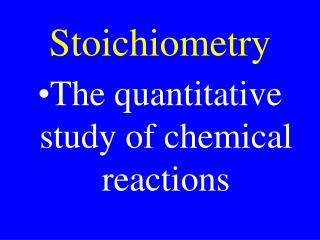DownloadDownload PresentationStoichiometry

# Stoichiometry

Télécharger la présentation## Stoichiometry

- - - - - - - - - - - - - - - - - - - - - - - - - - - E N D - - - - - - - - - - - - - - - - - - - - - - - - - - -
##### Presentation Transcript

1. Stoichiometry • The quantitative study of chemical reactions

2. Stoichiometric Steps • Set up & balance rxn • Change stuff given to moles • Change moles given to ask • Change what’s asked for to the proper unit

3. Step 1 • Determine products of a reaction if they are not given • Balance the reaction

4. Step 2 • Use molar conversion to change whatever is given to moles

5. Step 3 • Use the molar ratio from the balanced reaction to convert the moles of what is given to the moles of what’s asked for.

6. Step 4 • Use molar conversions to change the moles of what is asked for to the proper unit

7. Calculate the volume of NH3 formed at STP when 6.0 kg of H2 react with excess N2 to form NH3

8. Drill: Calculate the number of molecules of oxygen gas required to burn3.0 ng of C5H10O5

9. Chm II: Calculate the mass of Lead(II)iodide formed when 66.2 g of lead (II)nitrate is combined with excess potassium iodide

10. AP: Calculate the mass of solid product formed when 66.2 g of lead (II)nitrate is combined with excess potassium iodide solution

11. Chm II: Calculate the mass of MgCO3 formed when 18.4 g of MgBr2 is combined with a solution containing excess K2CO3.

12. Homework • Problem: 60 • Page 78

13. AP: Calculate the mass of solid product formed when 18.4 g of MgBr2 is combined with a solution containing excess K2CO3.

14. Chm II: Calculate the volume at STP of CO2 formed when 16.8 g of MgCO3 is decomposed creating CO2 & MgO.

15. AP: Calculate the volume at STP of gas formed when 16.8 g of MgCO3 is decomposed by heat.

16. Limiting Reactant • The reactant that gets used up • The reactant that determines the amount of product formed

17. Excess Reactant • The reactant that Does Not get used up

18. Stoichiometry with Multiple Reagents • Perform same steps for all reactants • Choose least amount of product

19. Calculate the mass of glucose (C6H12O6) formed when 880 g of carbon dioxide is combined with 720 g of water in photosynthesis

20. Chm II: Calculate the mass of BaSO4 formed when 104 g of BaCl2 is combined with 250 mL 1.00 M K2SO4

21. AP: Calculate the mass of solid formed when 104 g of BaCl2 is combined with 250 mL 1.00 M K2SO4

22. AP Drill: Calculate the mass of solid formed when 26.1 g of Ba(NO3)2 is combined with 25 mL 1.00 M K2SO4

23. Theoretical Yield • The amount determined through stoichiometry • The amount solved for on paper

24. Experimental Yield • The amount obtained in the lab • Actual yield

25. Percent yield % Yield = (Exp/Theo) x 100 %

26. 68 g NH3 was obtained in lab when 140 g of N2 reacts with excess H2. Calculate percent yield.

27. Drill: 510 g PH3 was obtained when 0.62 kg of P4 reacts with excess H2. Calculate percent yield.

28. Review HW • Problem 31 • on page 115

29. Determine the mass of PH3 formed at STP when 2.0 x 1027 molecules P4 reacts with 4.0 kg of H2:

30. Determine the volume of O2 released at STP when 32 kg of Fe2O3 is purified with an 80.0 % yield making Fe & O2

31. Calculate the volume of oxygen gas at STP required to burn 12 kg of erythrose (C4H8O4)

32. Calculate the mass of solid product formed when 41.6 g of barium chloride is added to 250 ml of 0.40 M potassium sulfate giving a 50.0 % yield:

33. Calculate the mass of solid product formed when 2.00 g of calcium bromide is added to 250 ml of 0.40 M sodium sulfate:

34. 4.61 g of lead(II)iodide was obtained when 66.2 g of lead(II)nitrate was added to 750 ml 0.10 M potassium iodide. Calculate the % yield & the molarity of all ions remaining in the solution.

35. 33.1 g of lead(II)nitrate was added to 1500 mL 0.10 M potassium iodide. Calculate mass of solid product formed.

36. An 30.0 g unknown sample (containing only C, H, & O) was burned in excess oxygen yielding 66 g CO2 & 36 g H2O. Calculate its empirical formula :

37. An 240 g unknown sample (containing only C, H, S, & O) was burned in excess oxygen yielding 352 g CO2, 144 g H2O, & 128 g, SO2. Calculate its empirical formula :